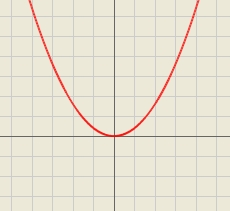# Consider

Consider all square prisms with a height of 10 cm.
If x is the measurement of the base edge, in cm, and y is the volume of the prism, in cm3. Graph the function

cm3Did you find an error or inaccuracy? Feel free to write us. Thank you!Tips to related online calculators
Looking for help with calculating roots of a quadratic equation?
Tip: Our volume units converter will help you with the conversion of volume units.

#### You need to know the following knowledge to solve this word math problem:

We encourage you to watch this tutorial video on this math problem: